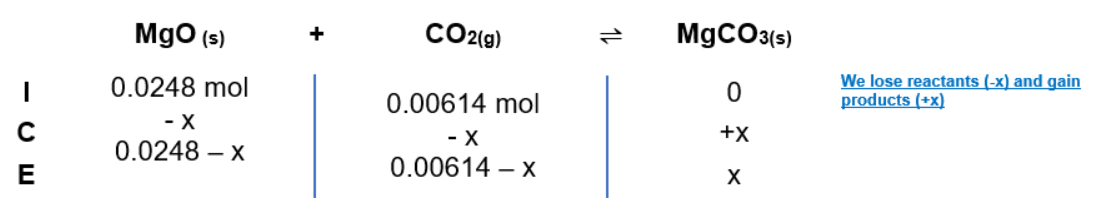# Problem: At 650 K, the reaction MgCO3(s) ⇌ MgO(s) + CO2(g) has Kp = 0.026. A 12.6 L container at 650 K has 1.0 g of MgO(s) and CO2 at P = 0.0260 atm. The container is then compressed to a volume of 0.600 L. Find the mass of MgCO3 that is formed.

###### FREE Expert Solution

Given reaction: MgCO3(s) ⇌ MgO(s) + CO2(g

Solve moles CO2:

nCO2 = 6.14 x 10-3 mol CO2

Solve moles MgO:

0.0248 mol MgO

ICE Chart(reverse reaction during compression)85% (304 ratings)###### Problem Details

At 650 K, the reaction MgCO3(s) ⇌ MgO(s) + CO2(g) has Kp = 0.026. A 12.6 L container at 650 K has 1.0 g of MgO(s) and CO2 at P = 0.0260 atm. The container is then compressed to a volume of 0.600 L. Find the mass of MgCO3 that is formed.

Frequently Asked Questions

What scientific concept do you need to know in order to solve this problem?

Our tutors have indicated that to solve this problem you will need to apply the ICE Chart concept. You can view video lessons to learn ICE Chart. Or if you need more ICE Chart practice, you can also practice ICE Chart practice problems.

What professor is this problem relevant for?

Based on our data, we think this problem is relevant for Professor Broering's class at CU.